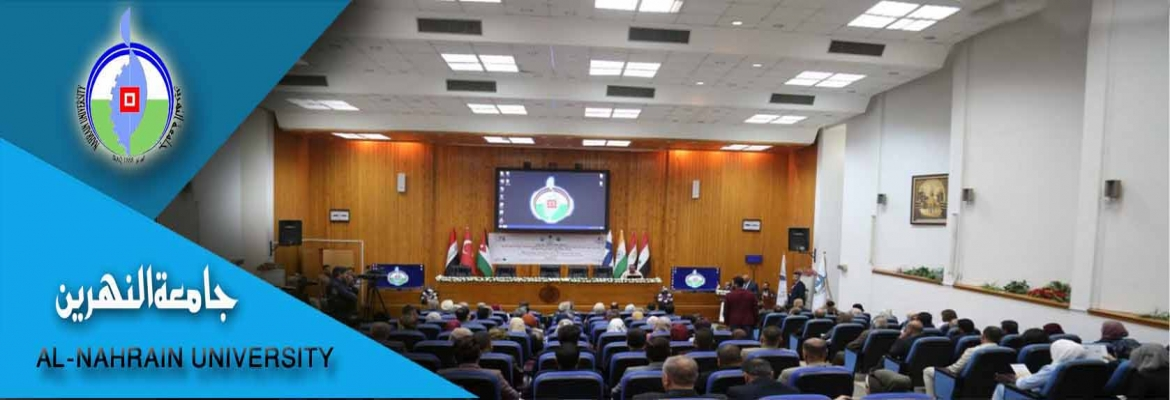# PREDICTION OF MASS TRANSFER COEFFICIENT UNDER TWO PHASE FLOW CONDITIONS USING TURBULENT DIFFUSION MODELS

number:
1841
Degree:
Author:
Supervisor:
Dr. Basim O. Hasan.
year:
2008

A theoretical study has been performed to analyze the mass transfer and shear stress in co-current annular,two phase gas-liquid (air-water), turbulent flow conditions in a horizontal pipe using eddy diffusivity concept. The mass transfer coefficient has been predicted and discussed for a wide range of liquid superficial Reynolds number (Re=2000 to 40000), gas superficial Reynolds number (Re=10000 to 80000), liquid Schmidt number (Sc temperatures (25oC - 60oLsgsL=100 to 3000), and liquid C). For the same ranges, the wall and interfacial shear stresses and friction factors are estimated using experimental and theoretical correlations proposed for two phase flow. The variation of eddy diffusivity is divided into two main regions, one in the liquid phase and the other in the gas phase. A new expression for eddy diffusivity is developed using three resistances in series. Using this expression an equation for calculating the mass transfer coefficient for a wide range of Re, Sc, and void fractions (or liquid layer thickness) is derived. Numerous previously proposed correlations are used to calculate the void fraction from fluids (air and water) velocities and their physical properties. The influence of liquid Resg, gas Resg, liquid Sc, and void fraction on the mass transfer coefficient, shear stresses, and friction factors is studied and discussed. In addition, the theoretical analysis included the estimation of shear stress and friction factor for stratified flow.  The analytical results are verified by comparison with the empirical mass transfer correlations obtained from diffusion controlled corrosion studies under two phase flow conditions by other workers.  The results showed that the eddy diffusivity is an efficient way to predict the mass transfer coefficient under annular two phase flow conditions.Also increasing ResL and Resg leads to increase of mass transfer coefficient but the influence of ResL is higher than that of Resg. The liquid Sc has a major effect on mass transfer coefficient while gas Sc has a minor effect. The ResL, Resg,and temperature affect the wall and interfacial shear stress and friction factor. Applying statistical analysis to the results, a correlation is obtained to predict mass transfer coefficient for the investigated range of Re and Sc.thesis.pdf

## اخبار كلية الهندسة

Education - This is a contributing Drupal Theme
Design by WeebPal.